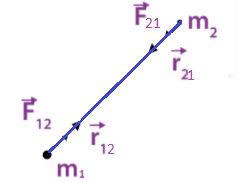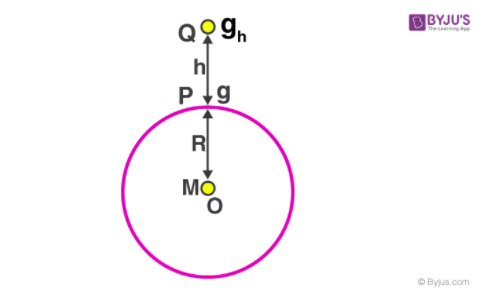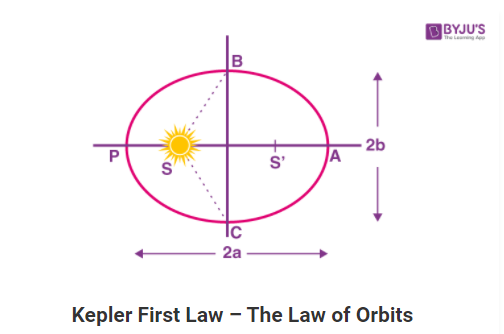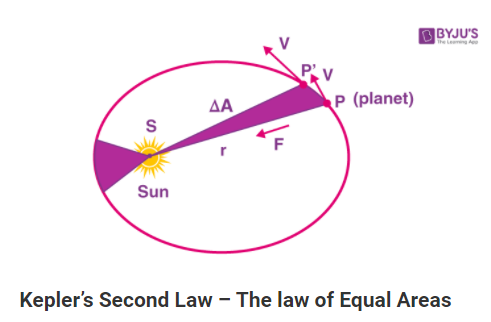# Gravitation Notes for IIT JEE

Gravitational force is one of the basic forces of nature. It is a force that acts between two bodies and it is always attractive in nature. Gravitational force is the force with which the objects are attracted to the centre of the earth. The motion of the planets and the moon are also governed by this force.

## Universal Law of Gravitation

Newton made a systematic study of the forces acting on the different particles and formulated his result. This is called the universal law of gravitation.

According to the law

“ The force of attraction between any two particles in the universe is directly proportional to the product of the masses and inversely proportional to the square of the distance between them.”

If the two masses m1 and m2 are at a distance of d. The force of attraction between them is

F = G(m1m2/d2)

G is the universal gravitational constant = 6.673 x 10-11 Nm2/kg2

Vector form of Newton’s law of Gravitation$\vec{F_{12}}=-\frac{Gm_{1}m_{2}}{r^{2}}\hat{r_{21}}$ ——-(1)

$\vec{F_{21}}=-\frac{Gm_{1}m_{2}}{r^{2}}\hat{r_{12}}$——–(2)

Since $\hat{r_{12}}=\hat{r_{21}}$

$\vec{F_{21}}=\frac{Gm_{1}m_{2}}{r^{2}}\hat{r_{21}}$

Therefore $\vec{F_{21}}=-\vec{F_{12}}$

## Acceleration Due to Gravity

The acceleration due to gravity is the rate of increase of velocity of a body falling freely towards the earth. Acceleration due to gravity does not depend on the shape, size and mass of the object. It is denoted by the letter g.

Relation between g and G

g = GM/R2

G = gravitational constant

M = mass of the earth

R = radius of the earth

The expression of acceleration due to gravity does not depend on the mass (m) of the object. If two bodies of two different masses are allowed to fall freely, they will fall with the same acceleration.

### Variation of Acceleration due to Gravity (g)

The value of g will differ as we move from place to place on the surface of the earth and as we move above and below the surface of the earth.

Variation of g with height$g’ = g(1-\frac{2h}{R})$

g’ = acceleration at the height h above the ground

h = height above the surface of the earth

g = acceleration due to gravity on the earth’s surface

R = radius of the earth

As height h increases, g’ decreases

Variation of g with depth

$g’ = g(1-\frac{d}{R})$

g’ = acceleration at the depth d below the surface of the earth

d = depth below the surface of the earth

g = acceleration due to gravity on the earth’s surface

R = radius of the earth

As depth d increases, g’ decreases

Variation of g with latitude

The earth rotates about its own axis with an angular velocity ω. The acceleration at a place with the latitude θ is

g’ = g – Rω2cos θ

The value of g increases as we move from the equator to the pole.

## Kepler’s Laws

Kepler’s first law (law of elliptical orbit): All the planets will revolve around the sun in an elliptical orbit with the sun at one focus.Kepler’s second law (law of areal velocities): The line drawn from the sun to any planet will cover an equal area of space in equal intervals of time.Kepler’s third law (law of time period): The square of the period of revolution of any planet about the sun is directly proportional to the cube of the mean distance from the sun.

## Gravitational Field

The gravitational field is the space around the mass or an assembly of masses over which it can exert a gravitational force on other masses.

Gravitational Field Strength or Gravitational Field Intensity

The gravitational field strength at any point in a gravitational field is given by the force experienced by the unit mass placed at that point. It is directed towards the particle producing the field.

$\vec{E}=\frac{\vec{F}}{m}$

## Gravitational Potential

At a point in a gravitational field, potential V is defined as the negative of the work done per unit mass in shifting a rest mass from some reference point to the given point.

V = – W/m

Gravitational potential due to a point mass

Suppose a point mass M is situated at a point O. The gravitational potential at a point P at a distance of ‘r’ from O is

V = – GM/r

Gravitational Potential of a Uniform Solid Sphere

Case 1: If the point ‘P’ lies inside the sphere (r < R)

V = -GM [(3R2 – r2)/2R2]

Case 2: If the point ‘P’ lies on the surface of the sphere (r = R)

V = -GM/R

Case 3: If the point ‘P’ lies outside the sphere (r ＞R)

V = -GM/R

Case 4: Gravitational potential at the centre of the solid sphere is

V = (-3/2) × (GM/R)

## Gravitational Potential Energy

Assuming the potential energy at infinity to be zero

U = – GMm/r

The above equation gives the potential energy of a particle of mass m separated from the centre of the earth by a distance of r.

Gravitational potential of a two-particle system

The gravitational potential energy of two particles m1 and m2 separated by a distance of r is

U = – Gm1m2/r

Gravitational potential of a three-particle system

$U = -\left ( \frac{Gm_{1}m_{2}}{r_{12}} +\frac{Gm_{1}m_{3}}{r_{13}}+\frac{Gm_{2}m_{3}}{r_{23}}\right )$

## Satellites

A satellite may be defined as a body that moves continuously around a comparatively large body. The satellite may be natural or artificial.

Natural Satellite:

The celestial body which revolves around the planet is called a natural satellite. For example, the moon is a natural satellite of the earth.

Artificial Satellite:

The human-made bodies that are placed in specific orbits and are made to revolve around the earth are called artificial satellites

Geostationary Satellite

A geostationary satellite will revolve around the earth with the same angular velocity and in the same direction as that of the earth around its axis.

### Escape Velocity

The minimum velocity of projection of a body for which it will escape the gravitational attraction of earth is known as escape velocity.

$V_{e}=\sqrt{\frac{2GM}{R}}=\sqrt{2gR}$

Substituting the value of ‘g’ and ‘G’ we get

ve = 11.2 km/s

### Orbital Velocity

$V_{0}=\sqrt{\frac{GM}{r}}$

If the satellite is at a height h above the surface of the earth, then r = R + h

$V_{0}=\sqrt{\frac{GM}{R+h}}$

Relation between Escape Velocity and Orbital Velocity

v0 =ve/√2 (if h < R)

### Time Period of Satellite

The time period of the satellite is the time taken by the satellite to complete one revolution around the earth.

Time period = Circumference of circular orbit/Orbital velocity

T = 2πr/v0

= 2π (R+h)/v0

But orbit velocity, $V_{0}=\sqrt{\frac{GM}{R+h}}$

T = [2π (R+h)]/ [√GM/R+h]

$T= \frac{2\pi (R+h)^{3/2}}{\sqrt{GM}}$

The time period of the satellite depends only on its height from the surface of the earth.

### Energy of Orbiting Satellite

Kinetic Energy Of Satellite

The satellite makes a pure rotational motion. In a pure rotation, the kinetic energy of the satellite is given by

$KE=\frac{1}{2}mv_{0}^{2}=\frac{1}{2}\frac{GMm}{r}=\frac{1}{2}\frac{GMm}{R+h}$

Potential Energy Of A Satellite

Potential energy is the energy possessed by the body in a particular position.

$U=-\frac{GMm}{r}=\frac{GMm}{R+h}$

Total Energy Of A Satellite

The total energy of the satellite is the sum of all energies possessed by the satellite in the orbit around the earth. As only the mechanical motion of the satellite is considered, it has only kinetic and potential energies.

Total energy of the satellite = kinetic energy of the satellite + potential energy of the satellite

E = KE + U

$E=-\frac{GMm}{2r}=\frac{GMm}{2(R+h)}$

## Binding Energy

The energy required to remove the satellite from its orbit to infinity is called the binding energy of the system.

Binding Energy of the satellite = -E

= GMm/2r

## Weightlessness

The weight of the body is due to the reactionary force applied to the body. If for a certain condition the reactionary force applied becomes zero then we will feel as though our weight is zero. This is called weightlessness. If the rope of a descending lift is broken then the people inside will feel weightless. This state will also be felt by the people in an artificial satellite.

If an artificial satellite of mass m is revolving around the earth in a circular orbit of radius R0. The necessary centripetal force is provided by the gravitational force between the satellite and the earth.

$\frac{GMm}{R_{o}^{2}}=\frac{mv^{2}}{R_{0}}$

$\frac{GM}{R_{o}^{2}}=\frac{v^{2}}{R_{0}}$ ———–(1)

Let m’ be the mass of the person inside the satellite

He will experience two forces:

The gravitational force = GMm’/R02

The reaction force F’ exerted by the earth on him

The net force (GMm’/R02 – F’) is balanced by the centripetal force m’v2/R0

(GMm’/R02 – F’) = m’v2/R0

(GM/R02 – F’/m’) = v2/R0———–(2)

From equation (1) and (2) we have

(GM/R02 – F’/m’) = GM/R02

or F’ = 0

Thus, the reaction force exerted by the article satellite on the person inside the satellite is zero.

### Important Points To Remember

• The law of gravitation is applicable to all bodies irrespective of their size, shape and position.
• The gravitational force between the sun and the planets provides the necessary centripetal force for orbital motion.
• The gravitational force is always attractive.
• The acceleration due to gravity decreases as the height or the depth increases from the surface of the earth.
• Kepler discovered the three laws of planetary motion.
• Kepler’s laws are applicable not only to planets but also to other satellites in orbit around any body.
• Escape velocity is the minimum velocity with which an object must be projected to escape the gravitational attraction of the earth.

## Solved Examples

(1)Two balls, each of radius R, equal mass and density are placed in contact, then the force of gravitation between them is proportional to

a) F ∝ 1/R2

b) F ∝ R

c) F ∝ R4

d) F ∝ 1/R

Solution:

Distance between the centre of the two balls = R + R = 2R

Mass of the ball m = ρ x (4/3) πR3

Gravitational force F = GMm/r2

Since M = m

F = Gm2/(2R)2

= G (ρ x (4/3) πR3)/4R2

⇒ F ∝ R4

(2) If M is the mass of the earth and R its radius, the ratio of the gravitational acceleration and the gravitational constant is

a) R2/M

b) M/R2

c) MR2

d) M/R

Solution:

Gravitational acceleration, g = GM/R2

Here G is the gravitational constant

g/G = M/R2

(3) The weight of a body on the surface of the earth is 12.6 N. When it is raised to a height half the radius of the earth, its weight will be

a) 2.8 N

b) 5.6 N

c) 12.5 N

d) 25.2 N

Solution:

Given,

Weight of the body on the surface of the earth, W0 = 12.6 N

h = R/2

Weight of the body at a height h, W’ = W0 (R/R+h)2

W’ = 12.6 (R/R+ R/2)2

W’ = 12.6 x (4/9) = 5.6 N

(4) The acceleration due to gravity becomes (g/2) at a height equal to (g = acceleration due to gravity on the surface of the earth)

a) 4R

b) R/4

c) 2R

d) R/2

Solution:

The acceleration due to gravity, g = GM/R2

At a height h above the surface of the earth, the acceleration due to gravity will be

g’= GM/(R+h)2

$\frac{g}{g’}=\left ( \frac{R+h}{R} \right )^{2}$

= (1 + h/R)2

$\frac{g’}{g}=\left ( 1+\frac{h}{R} \right )^{-2}=\left ( 1-\frac{2h}{R} \right )$ (when h ＜＜R)

g’ = g/2

Therefore, $\frac{g/2}{g}=\left ( 1-\frac{2h}{R} \right )$

(2h/R) = ½

Or h = R/4

(5) The ratio of acceleration due to gravity at a height 3R above the earth’s surface to the acceleration due to gravity on the surface of the earth is ( R = radius of the earth )

a) 1/9

b) ¼

c) 1/16

d) ⅓

Solution:

The acceleration due to gravity on the surface of earth g = GM/R2

The acceleration due to gravity at a height 3R from the surface of earth, g’ = GM/(R + 3R)2

g’/g = [GM/(R + 3R)2] x R2/GM

= R2/16R2

g’/g= (1/16)

## Practice Problems

(1) Imagine a new planet having the same density as that of the earth but it is 3 times bigger than the earth in size. If the acceleration due to gravity on the surface of the earth is g and that on the surface of the new planet is ‘g’, then

a) g’ = 2g

b) g’ = 3g

c) g’ = 4g

d) g’ = 5g

(2) The mass of a planet is six times that of the earth. The radius of the planet is twice that of the earth. If the escape velocity from the earth is v, then the escape velocity from the planet is

a) √3 v

b) √2 v

c) v

d) √5 v

e) √12 v

(3) A body is orbiting very close to the earth’s surface with kinetic energy KE. The energy required to completely escape from it is

a) KE

b) 2KE

c) KE/2

d) 3KE/2

(4) A solid sphere of mass M and radius R has a spherical cavity of radius R/2 such that the centre of the cavity is at a distance of R / 2 from the centre of the sphere. A point mass m is placed inside the cavity at a distance of R / 4 from the centre of the sphere. The gravitational pull between the sphere and the point mass m is

a) 11 GMm/R2

b) 14 GMm/R2

c) GMm/2R2

d) GMm/R2

(5) Acceleration due to gravity is g on the surface of the earth. Then, the value of the acceleration due to gravity at a height of 32 km above the earth’s surface is (assume the radius of the earth to be 6400 km)

a) 0.99 g

b) 0.8 g

c) 1.01 g

d) 0.9 g

### Why is the gravitational potential energy always negative?

The gravitational force is always attractive because of which the gravitational potential energy is always negative.

### Kepler’s Second Law is based on which fundamental law of Physics?

Kepler’s Second Law is based on the Law of conservation of angular momentum.

### Mention two characteristics of gravitational force.

(i) The gravitational force is a central force and it is always attractive.
(ii) It follows the inverse square law

### State two essential requirements of a geostationary satellite.

(i) The period of rotation of the geostationary satellite must be the same as the earth’s rotation about its axis.
(ii) A geostationary satellite will revolve around the earth with the same angular velocity and in the same direction as that of the earth around its axis.

### Why does a body reach the ground quicker at poles than at the equator when dropped from the same height?

The acceleration due to gravity is more at the poles than at the equator. So, when an object is dropped from the same height, the object reaches the ground faster at the poles than at the equator. If the initial velocity and the distance travelled by the object are the same then the time taken to reach the ground will be less if the acceleration due to gravity is more.